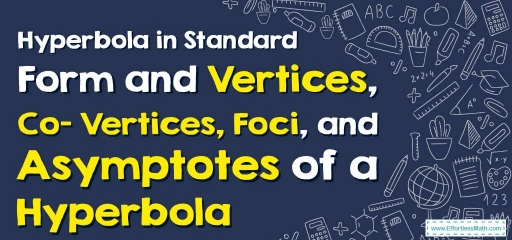# Hyperbola in Standard Form and Vertices, Co– Vertices, Foci, and Asymptotes of a Hyperbola

Hyperbole is determined by the center, vertices, and asymptotes.The standard forms for the equation of hyperbolas are:

$$\frac{(y-k)^2}{a^2}-\frac{(x-h)^2}{b^2}=1$$ and $$\frac{(x-h)^2}{a^2}-\frac{(y-k)^2}{b^2}=1$$

The information of each form is written in the table below:

### Hyperbola in Standard Form and Vertices, Co– Vertices, Foci, and Asymptotes of a Hyperbola – Example 1:

Find the center and foci of $$x^2+y^2+8x-4y-44=0$$

Solution:

To rewrite in standard form, first add $$44$$ to both sides: $$x^2+y^2+8x-4y=44$$

Group $$x$$ -variables and $$y$$ -variables together: $$(x^2+8x)+(y^2-4y)=44$$

Convert $$x$$ and $$y$$ to square form: $$(x^2+8x+16)+(y^2-4y+4)=44+16+4 → (x+4)^2+(y-2)^2=64$$

Divide by $$64$$: $$\frac{(x+4)^2}{64}-\frac{(y-2)^2}{64}=1$$

Then: $$(h, k)=(-4, 2), a=8, b=8,$$ and center is $$(-4, 2)$$

Foci: $$(-4, 2+c), (-4,2-c)$$

Compute $$c: c=\sqrt{8^2+8^2}=8\sqrt{2}$$ then: $$(-4, 2+8\sqrt{2}), (-4, 2-8\sqrt{2})$$

### What people say about "Hyperbola in Standard Form and Vertices, Co– Vertices, Foci, and Asymptotes of a Hyperbola - Effortless Math: We Help Students Learn to LOVE Mathematics"?

No one replied yet.

X
30% OFF

Limited time only!

Save Over 30%

SAVE $5 It was$16.99 now it is \$11.99